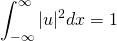# In the BeginningContents

In the beginning there was continuous flow, and then Max Planck came along and proposed quantization. Quantization basically just means, that instead of being continuous, things such as EM radiation, can only exist in multiples of certain values. It’s a little bit like having a tube of smarties. The whole tube represents a beam of light. Inside it you have the smarties. You can split the tube, so you can have less smarties in there, or you can get another tube and have smarties, but you have to have a whole number of smarties, because they can’t be split (if anyone e-mails me suggesting I squash/crush/split a smartie, I will hunt them down and make them pay!).

Planck came to this conclusion when working on the “Ultraviolet Catastrophe”. According to classical electromagnetism, the number of ways an electromagnetic wave can vibrate a in a 3-dimensional cavity, per unit frequency, is proportional to the square of the frequency. This means that the power you would get out per unit frequency should follow the Rayleigh-Jeans law, which means that the power would be proportional to the frequency squared. So if you put the frequency up higher and higher the power would be unlimited. Planck said that electromagnetic energy did not follow the classical description. He said that it could only be emitted in discrete packets of energy proportional to the frequencyor sometimes written aswhere(pronounced “h bar”) is h/(2). These equations mean the the radiation eventually goes to zero at infinite frequencies, and the total power is finite. Planck called these packets of energy “Quanta”. The value of h isJ·s and the value ofisJ·s.

Quantum behaviour differs from classical behaviour because h is not equal to 0

# Let There be Little Packets of Light

If you shine a light onto a metal surface for long enough the surface will heat up. This must mean that the light is transferring energy to the metal, so in theory it is possible that if you shone a light on a surface for long enough, enough energy would be transferred to liberate an electron from an orbit. Even with a weak light you should be able to wait long enough for the energy to build up and an electron to be emitted. So physicists tried the experiment. It failed miserably. For some metals specific light would cause electron emissions, for other metals the same light source wouldn’t, no matter how long it was left. And it was found that the electrons came out with higher energies depending on the colour of the light, not the intensity.

The problem of the photoelectric effect was solved in 1905 by Einstein, and was what he won the Nobel Prize for in 1921. Einstein applied Plancks theory of Quantization to light and said that the light is not a continuous stream of energy but rather loads of little packets of a certain energy value that depended on its wavelength. This explained why no matter how long you left the light on the surface there would be no emission unless the individual photons had enough energy. This also explained why different colours gave the emitted electrons different energy values. The energy was shown to be related to wavelength by Plancks equation. Einstein also showed that the energy of the emitted electrons would be equal towhere Φ is the energy needed to get the electron from inside the metal to just outside the surface, and is called the “Work Function”.

# Schrödinger Equation

We start off with a standard equation for a wave.

(1)means that what this wave looks like depends on position () and time (). The description is set out in complex number form and can be displayed with an Argand diagram (For more info see here). This wave is a solution of the Wave Equation, and what we want to see is if the wave equation can be used to describe matter waves. The wave equation is

(2)What this equation is saying is that, if you partially differentiate your wave,, with respect totwice, it will equal the partial differential of your wave with respect totwice, multiplied by a constant, which in this case is.

So now we need to see if it will work, so first we take our wave (1) and differentiate it twice with respect to(If you are unsure how to do this see here for help). So differentiating twice gives.You may be wondering why I’ve changed the original equation whilst doing the differentiations. Originally we hadand now we have. This is just a maths trick you can do to exponential powers and I personally think it makes the differentiation easier. We now differentiate the wave twice with respect to time to getWe can now substitute these two results into equation (2) to giveConveniently the minus signs, the‘s and the squares cancel to give us

(3)Now, if we take the 2 base quantum formulas from the first section

(4)(5)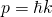and try and substitute (3) into them we get a problemHoweverfor matter. For non-relativistic matter the relationship between energy and momentum obeys the following law

(6)So it looks like we have a problem. The Wave Equation (2) doesn’t work for matter. One way to try and get it to work is to say that instead of, what if we tried to get it so it was? To do this we would need a wave equation that was differentiated twice withand only once with. Also if we replace the constant we can make life easier for ourselves. So lets tryas our new wave equation. We have now changedto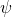as this will be the equation that works andis the common symbol used for quantum mechanical waves, the equation foris the same as for. So if we now do the differentiationWhich when we put back in our new wave equation gives usWhich is the relationship betweenandwe were after. So now we can rearrange forand substitute into equations (4) to getWe can now chose our constantasso we getWhich is correct!! So far so good, our new wave equation for matter gives us the correct energy. So let’s put the constant back inAnd now once again rearrange to get it in a nicer looking form,We’re nearly there now. The equation is almost complete. However when we solve it for the energy of a particle we getbut sometimes a particle can get energy from its surroundings, for example if it was in a potential, so we have to make one slight adjustment to account for all of the particles possible energiesWhich means our wave equation becomesThis is called the One Dimensional Time Dependent Schrödinger Equation

## Time Independence

In most cases you’ll learn about involving matter waves like electrons, the potentials they’re in don’t really depend on time, they don’t suddenly change shape after so many seconds. If this is the case (and most of the time it is) then we can use the Separation of Variables method on the Schrödinger Equation.

First thing we do is assume that thecan be split into two functions, one that only depends onand one that only depends on, like soYou then get your Schrödinger Equation and wherever there is ayou just replace it with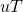, so you getNow, you divide by, you get rid of the one on the left as that differential doesn’t depend on, and if you divide through byyou get rid of theon the right as that differentiation doesn’t depend on. So you get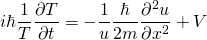Now lets saychanged, that would mean that the left hand of the equation would now have a different value, however asis independent ofthe right hand side of the equation wouldn’t change. This would cause an error. The two sides of the equation were equal before, now one side has changed and they still have to be equal. To get around this problem you set both sides equal to a constant, in this case we shall call it. So now we have two separate equations,

(7)(8)We have already prefaced that we are only interested in cases where time has no affect on the potential so we can ignore equation (7) and just use equation (8), which is our One Dimensional Time Independent Schrödinger Equation. In the case of a free particleso the solution to the time independent equation (8) becomeswhereThis means the wavefunction has the formWhich is of an identical form to equation (1), provided that the constantis the total energy of the system, which is a good thing. This means that provided we know the energy of the system, we can work out a solution to the wave equation. Also, provided the energy remains constant,has no effect on, sois just the same as, so then we can write